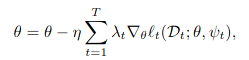# Weighted sample losses

I am working on a project where I have to calculate the losses for each individual sample t. These losses are multiplied by an individual sample weight lambda. I would now like to calculate the gradients for each individual loss before multiplying them with the given sample weight. However, I am not sure about the dimensions. For one sample loss, calculating the gradients, in my case, results in 14 tensors with individual length. Should I just multiply each individual scalar in the resulting gradients with the respective weight?I think you do not need to deal with gradient calculations.
If you multiply the `lambda`s to the respective individual losses and sum/average it up, the gradient calculation will be handled accordingly by PyTorch.

Similar to the below code:

``````criterion = torch.nn.MSELoss(reduction='none')
...

# lambda = (b,), pred = (b, d), target = (b, d)
loss = lambda * criterion(pred, target)
loss = loss.mean()  # or sum()
loss.backward()
``````

Thanks for your answer! But wouldn’t that result in taking the gradients of the weighted losses? Since the equation asked for the gradients of the losses which are then weighted by lambda. Does it even matter whether I first multiply by the weights and then calculate the gradients or the other way around?

No. It doesn’t matter whether you multiply the loss by `lambda`s (weighted loss) or calculate the gradients first & then multiply the `lambda`s.

As I understand, `lambda`s are constant here. Taking the gradient of weighted loss is same as multiplying the `lambda`s after taking the gradient.